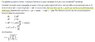# Confusion about the derivation of the speed of sound

• snoopies622
snoopies622
I've having trouble understanding a derivation of the speed of sound waves, which is actually similar to another derivation I found a couple days ago.

Let's suppose the sound is moving through water in a long cylindrical horizontal pipe. The premises of the derivation are

1.) For a given cylindrical slice of water of thickness $\Delta x$, the net horizontal (direction of the wave motion) force acting on the water is proportional to the hoizontal pressure gradient times $\Delta x$, so $$\rho \frac {dv}{dt} = \frac {- \partial P}{\partial x}$$
2.) the mass flux through any infinitely thin cylindrical slice is constant, or
$$\frac {d ( \rho v) }{dt} =0$$

And from these premises one can arrive at $$v^2 = \frac {dP }{d \rho }$$.

What I don't understand is why the second premise is true, since neither the water density nor water speed is constant. Or perhaps I don't understand the second premise: Is it supposed to be for an infinitely thin slice, or for a cylinder of thickness $\Delta x$, or something else?

Thanks.

snoopies622
For the Wikipedia entry "Speed of sound" they refer to "the two ends of the tube", which seems to me to be a very strange body of fluid to be looking at when the wave itself can be contained within a small fraction of that length.

#### Attachments

•wave-speed.jpg
42.7 KB · Views: 73
Arjan82
What you describe in words as equation 2) would be more like ##d(\rho v) / d x##. But what you state mathematically is that the mass flux doesn't change in time at any point. That is of course weird. Furthermore, these are all partial derivatives since ##v## and ##\rho## are dependent on both time and location. It is however close-ish to the linearized continuity equation:
$$\frac{\partial\tilde{\rho}}{\partial t} + \rho_0 \frac{\partial \tilde{v}}{\partial x} = 0$$
If you write ## v = \tilde{v} ##, ##\rho = \rho_0 + \tilde{\rho}## and ##p = p_0 + \tilde{p}## where all quantities with a tilde are small amplitude disturbances. I.e. you can neglect second order terms. This equation simply states that no mass is destroyed, i.e. a density change is compensated with an influx of mass (no 'gaps' in the flow field).

From this equation and your first equation you can derive the wave equation and speed of sound as you stated.

•vanhees71
snoopies622
Ah, the continuity equation! Thanks Arjan82, I don't know why neither essay mentioned that. I think that will completely solve my problem.

Gold Member
2022 Award
I'm puzzled by the derivation in the attachment to #2 too. I think the standard derivation (e.g., Landau Lifshitz vol. 6) is more transparent. All you need to know is that assuming a perfect fluid implies that the thermodynamic changes at any point in the fluid are adiabatic (at constant entropy).

You get sound waves as small perturbations around the static state of the fluid, i.e.,
$$\rho=\rho_0 + \delta \rho, \quad P=P_0 + \delta P, \quad \vec{v}=\delta \vec{v}.$$
Then take Euler's equation (just Newton's law ##F=m a## for an ideal-fluid element):
$$\rho [\partial_t \vec{v} + (\vec{v} \cdot \nabla) \vec{v}]=-\vec{\nabla} P.$$
Now since ##\vec{v}=\delta \vec{v}## we can neglect the 2nd term at the left-hand side and use that ##P_0=\text{const}##, leading to
$$\rho_0 \partial_t \delta \vec{v}=-\vec{\nabla} \delta P. \qquad (1)$$
The continuity equation (mass conservation law) reads
$$\partial_t \rho+\vec{\nabla} \cdot (\rho \vec{v})=0.$$
Now expanding up to the first order in ##\delta \rho## and ##\delta \vec{v}## you get
$$\vec{\nabla} \cdot (\rho \vec{v})=\vec{v} \cdot \vec{\nabla} \rho + \rho \vec{\nabla} \cdot \vec{V} = \rho_0 \vec{\nabla} \cdot \delta \vec{v}.$$
Thus the continuity equation simplifies to
$$\partial_t \delta \rho + \rho_0 \vec{\nabla} \cdot \delta \vec{v}=0.$$
So taking the divergence of Eq. (1) and using this equation you get
$$\rho_0 \partial_t \vec{\nabla} \cdot \delta \vec{v}=-\partial_t^2 \delta \rho=-\Delta \delta P. \qquad(2)$$
Now you need an equation of state, i.e., ##P=P(\rho)##, where you have to assume the entropy to be constant. Given that equation of state, you get
$$\delta P = \left (\frac{\partial P}{\partial \rho} \right)_{S,0} \delta \rho=:c^2.$$
The notation of the partial derivative means that you have to take the derivative at constant ##S## and for the thermodynamic quantities of the unperturbed fluid in thermal equilibrium. So plugging this into (2) you find
$$\partial_t^2 \delta \rho -c^2 \Delta \delta \rho=0,$$
which is a wave equation for a wave with phase velocity ##c## which thus is the sound velocity.

•Arjan82
snoopies622
That is more transparent, thanks vanhees71!

TOAsh2004
Just looked at this derivation in Landau & Lifshitz again and wondered why the equation of state looks like $$\delta P = \left (\frac{\partial P}{\partial \rho} \right)_{S,0} \delta \rho$$ Sure, the entropy is constant, but why can you assume the pressure to be only dependent on the density, and not on the temperature, which is surely not constant when the wave passes through.

$$\mathrm{d} U = T \mathrm{d} S - P \mathrm{d} V = T \mathrm{d} S+P/\rho^2 \mathrm{d} \rho.$$
$$P=\rho^2 (\partial_{\rho} U)_{S}.$$
•